# Exploratory Data Analysis on Iris dataset

By Vineeth Kumar Category Data Science Reading time 15-20 mins Published on Jan 3, 2021

What is Meant by Iris Dataset?

Being able to implement a data science project is more rigorous than it seems. Data scientists examine every step carefully to ensure that there are no anomalies or errors present. This is why scientists use Exploratory Data Analysis (EDA), which improves the accuracy of results.

EDA helps data scientists find errors, and missing values, understand different variables, and identify key patterns in data. It is extremely important for organizations for gaining better insights and conclusions from a dataset.

The Iris dataset is a set of information that displays the characteristics of different statistical models. It contains data on the Iris species of flower. The Iris flower dataset is a well-known dataset with multiple variables. It is specifically designed for testing different machine learning algorithms.

## What is EDA?

For a budding data scientist, EDA can help perform proper data analysis. EDA will help you extract and analyze information about the data before jumping to conclusions.

Exploratory data analysis aka EDA is a crucial process where we perform early data investigations. This process helps discover patterns, spot anomalies, test hypotheses and check assumptions with the help of summary statistics and graphical representations.

Exploring and comparing a data set with multiple exploratory techniques is a good practice. After the Iris dataset exploratory data analysis, you will get confidence in your data to the point where you're ready to implement a machine learning algorithm.

The Iris dataset EDA is helpful in selecting the feature variables that will be used later for machine learning.

## What is Iris Dataset?

The Iris dataset contains three different flower species (classes) of the Iris family, which are

• Iris setosa

• Iris versicolor

• Iris virginica

In the Iris dataset, each class contains 5 distinct features, namely Petal Width, Petal Length, Sepal Width, Sepal Length, and Species Type.

The Iris dataset is a basic function in data science which is why it is often referred to as the 'Hello World' of data science.

The main objective of this dataset is to classify a new flower having 4 unique features belonging to one of the three classes.

We will perform Exploratory Data Analysis (EDA) on the Iris dataset to find out meaningful patterns.

You can download the Iris dataset from Kaggle and start using it. The dataset contains 150 data points.

## EDA on Iris Dataset:-

First, load the Iris dataset CSV file obtained above using the Pandas library. Then convert the dataset into a data frame. We will use the same data frame object (iris_data) for the Iris dataset analysis.

### Importing relevant libraries:-

• import pandas as pd

• import numpy as np

• import seaborn as sns

• import matplotlib.pyplot as plt

• %matplotlib inline

• iris_data()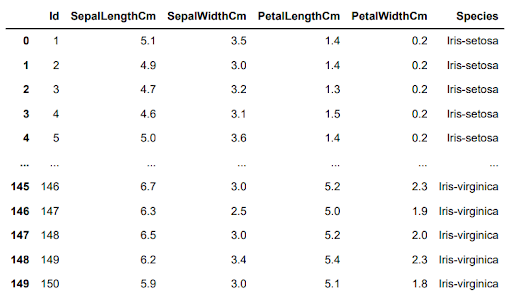We will get the shape of the Iris dataset using the shape parameter.

• iris_data.shape

(150,5)

### Analyzing the data:-

• iris_data.info() #provides information about the content of the dataset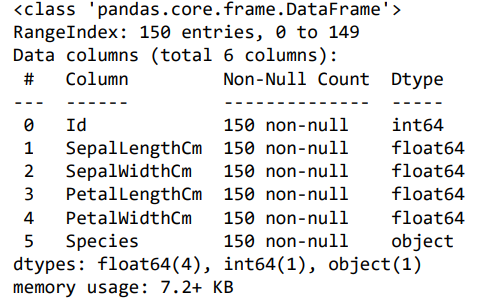#### Data insights:

1. There are no Null Entries in any of the columns.

2. There are four numerical columns.

3. There is only a single column of category type.

### Statistical analysis:-

• iris_data.describe()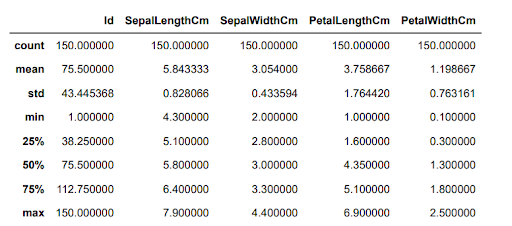#### Data insights:

We obtain the mean, minimum, and maximum values and the standard deviation for each feature.

### Dropping column:-

Here we are removing and dropping the unwanted columns.

iris_data = iris_data.drop('Id',axis = 1)

### Detecting duplicate data:-

• iris_data[iris_data.duplicated()]

#### Output:-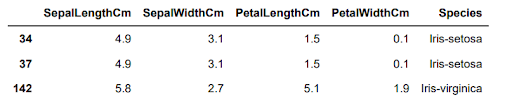The output displays 3 duplicate values. Because of these duplicates, we will determine if the Iris Dataset for each species is balanced in no or no's.

• iris_data['Species'].value_counts() # to check the balance

#### Output:-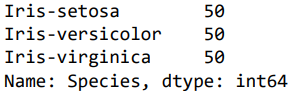As a result, we should not delete the data. Because doing so may cause an unbalance in the data sets, making them less helpful for meaningful insights.

### Species count:-

• plt.title('Species Count')

• sns.countplot(iris_data['Species'])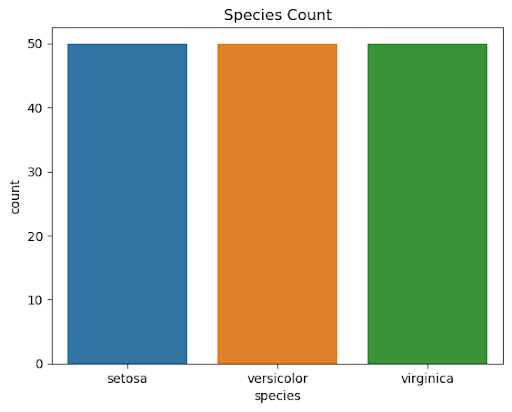#### Data insight:-

1. This illustrates how well-balanced the species are.

2. Each species (Iris virginica, setosa, and versicolor) has a count of 50.

## Univariate analysis:-

#### Comparison of different species depending on sepal width and length.**

• plt.figure(figsize=(8,6))

• plt.title('Comparing different species based on their sepal length and width')

• sns.scatterplot(iris_data['SepalLengthCm'],iris_data['SepalWidthCm'],hue =iris_data['Species'],s=50)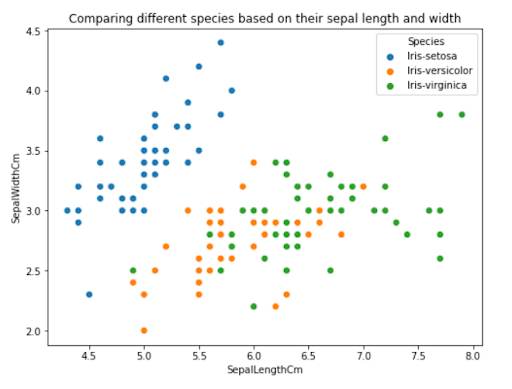#### Data insight:-

1. Iris Setosa has shorter sepals but wider petals.

2. Versicolor is almost in the center in both length and breadth.

3. Virginica has longer sepals and narrower sepals.

Comparison of different species depending on petal width and length.

• plt.figure(figsize=(8,6))

• plt.title('Comparing different species based on their petal length and petal width')

• sns.scatterplot(iris_data['PetalLengthCm'], iris_data['PetalWidthCm'], hue = iris_data['Species'], s= 50)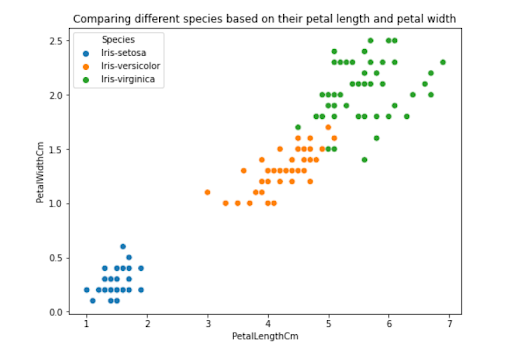#### Data insight:-

1. Setosa has the shortest petal length and breadth.

2. Petal length and breadth are normal for the Versicolor species.

3. Virginica species have the maximum petal length and breadth.

## Bi-variate analysis:-

• plt.figure(figsize=(10,9))

• sns.pairplot(iris_data,hue="Species",height=4)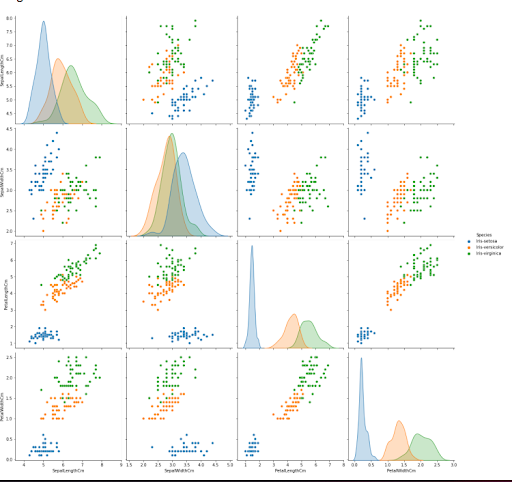#### Data insight:-

1. There is a strong correlation between columns for petal length and width.

2. Setosa has short petal width and length.

3. Setosa has a wide sepal and a short length.

4. Versicolor has standard petal width and length.

5. Virginica has long and wide petals.

6. Versicolor's sepal dimensions have average values.

7. Virginica has a narrow breadth but a long sepal length.

### Examining the correlation:-

• plt.figure(figsize=(5,5))

• sns.heatmap(iris_data.corr(),annot=True)

• plt.plot()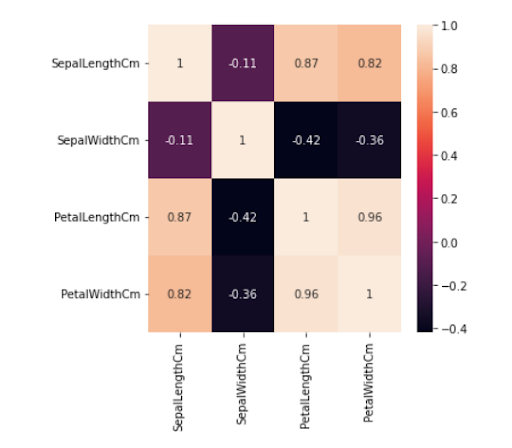#### Data insight:

1. Sepal Length and Width traits are marginally correlated with each other.

### Examining each species' mean and median values:-

• iris_data.groupby('Species').agg(['mean', 'median'])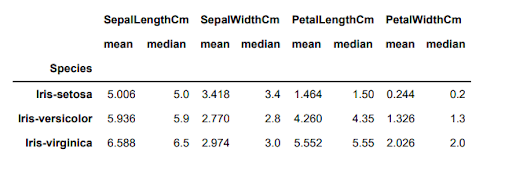## Use of box plots and violin plots to depict the distribution, mean, and median:-

### Box plots to learn about distribution-

Use a boxplot to observe how the categorical feature "Species" is divided in relation to the remaining four input variables.

• fig, axes = plt.subplots(2, 2, figsize=(10,9))

• sns.boxplot( y="PetalWidthCm", x= "Species", data=iris_data, orient='v' , ax=axes[0, 0])

• sns.boxplot( y="PetalLengthCm", x= "Species", data=iris_data, orient='v' , ax=axes[0, 1])

• sns.boxplot( y="SepalLengthCm", x= "Species", data=iris_data, orient='v' , ax=axes[1, 0])

• sns.boxplot( y="SepalWidthCm", x= "Species", data=iris_data, orient='v' , ax=axes[1, 1])

• plt.show()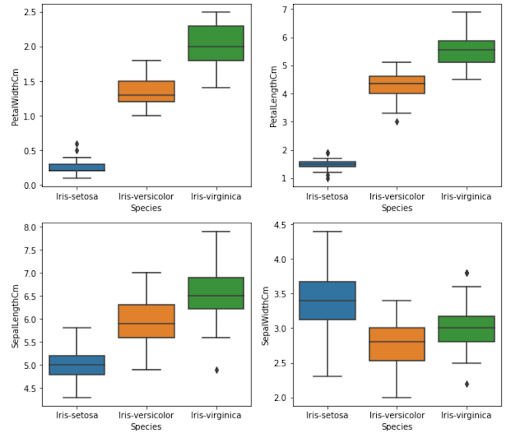#### Data insight:-

1. Setosa has fewer features and is less dispersed.

2. Versicolor is dispersed evenly and has average characteristics.

3. Virginica has a huge variety of qualities and characteristics and is widely dispersed.

4. Each plot clearly shows the mean/median values for several characteristics (sepal length & width, petal length & width)

### Violin plot for distribution analysis-

The violin plot depicts the species' density of width and length. The narrower portion indicates lower density, and the bigger portion indicates more density.

• fig, axes = plt.subplots(2, 2, figsize=(10,9))

• sns.violinplot( y="PetalWidthCm", x= "Species", data=iris_data, orient='v' , ax=axes[0, 0],inner='quartile')

• sns.violinplot( y="PetalLengthCm", x= "Species", data=iris_data, orient='v' , ax=axes[0, 1],inner='quartile')

• sns.violinplot( y="SepalLengthCm", x= "Species", data=iris_data, orient='v' , ax=axes[1, 0],inner='quartile')

• sns.violinplot( y="SepalWidthCm", x= "Species", data=iris_data, orient='v' , ax=axes[1, 1],inner='quartile')

• plt.show()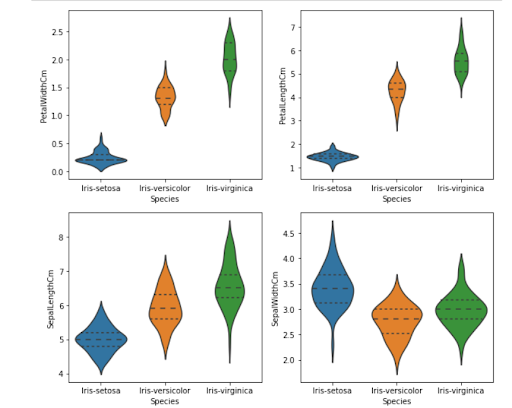#### Data insight:

1. Setosa has a lower dispersion density of petal width and length.

2. Versicolor is dispersed evenly and has average traits of petal length and breadth.

3. Virginica is widely dispersed, with sepal width and length showing a vast number of values and characteristics.

4. High-density values represent mean/median values. According to the table, Iris Setosa has the maximum density at 5.0 cm (sepal length characteristic), which is also the average value (5.0).

## Plotting the histogram and probability density function (PDF)

Plot the probability density function (PDF) using the variable as an individual feature on the X-axis. On the Y-axis, we plot its histogram and the associated kernel density.

plt.figure(figsize=(5,5))
plt.show()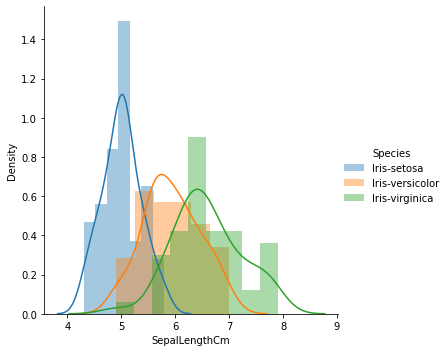Figure-1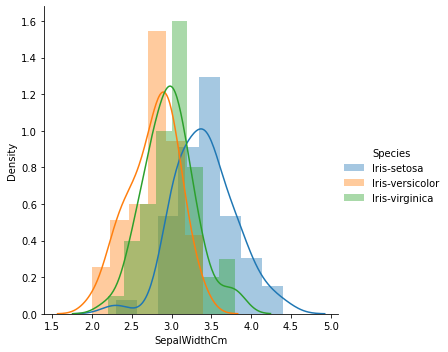Figure-2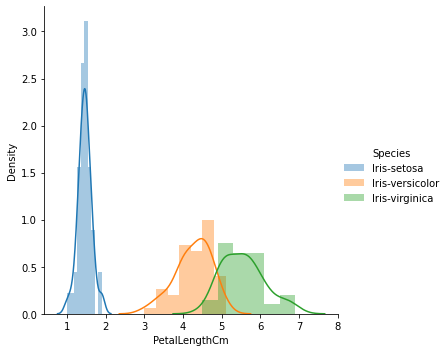Figure-3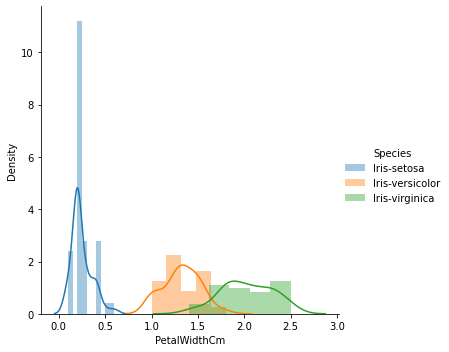Figure-4

#### Data insight:

• Figure 1 demonstrates that there is a substantial degree of overlap between the species in terms of sepal length, indicating that it is ineffective as a classification characteristic.

• Figure 2 reveals that there is significantly more overlap between the species on sepal width, indicating that it is ineffective as a classification characteristic.

• Figure 3 demonstrates that petal length is a useful classification characteristic since it clearly distinguishes across species. The overlap is little (between Versicolor and Virginica), while Setosa is well separated from the other two.

• Figure 4 demonstrates that petal width is a useful classification characteristic. The overlap is much smaller (between Versicolor and Virginica), while Setosa is well separated from the other two.

We will use the petal length as a classification characteristic from figure 3 to differentiate among the species.

## Plotting different classes of the target variable

iris_setosa = iris_data.loc[iris_data["Species"] == "Iris-setosa"];

iris_virginica = iris_data.loc[iris_data["Species"] == "Iris-virginica"];

iris_versicolor = iris_data.loc[iris_data["Species"] == "Iris-versicolor"];

plt.plot(iris_setosa["PetalLengthCm"],

np.zeros_like(iris_virginica["PetalLengthCm"]), 'o')

plt.plot(iris_versicolor["PetalLengthCm"], np.zeros_like(iris_virginica["PetalLengthCm"]), 'o')

plt.plot(iris_virginica["PetalLengthCm"], np.zeros_like(iris_virginica["PetalLengthCm"]), 'o')

plt.grid()

plt.show()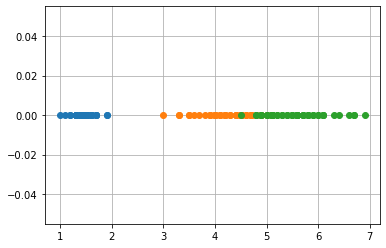#### Data insight:

1. Iris Setosa's pdf curve ends around 2.1.

2. If the petal length is greater than 2.1, the species is Iris Setosa.

3. The intersection point of the pdf curves of Versicolor and Virginica is approximately 4.8.

4. If the petal length is greater than 2.1 and less than 4.8, the species is Iris Versicolor.

5. If the petal length exceeds 4.8, the species is Iris Virginica.

## Pair Plot:-

A pair plot allows us to see both the distribution of single variables and the relationships between two variables. For example, let's say we have four features 'sepal length', 'sepal width', 'petal length', and 'petal width' in our Iris dataset. In that case, we will have 4C2 plots i.e. 6 unique plots. The pairs, in this case, will be :

• Sepal length, sepal width

• petal length, petal width

• sepal length, petal Width

• Petal length, sepal width

• Petal length, sepal length

• Petal width, sepal width

So, here instead of trying to visualize four dimensions which is not possible. We will look into 6 2D plots and try to understand the 4-dimensional data in the form of a matrix.

### 1st plot

sns.set_style("whitegrid");

plt.show()

### 2nd plot

sns.set_style("whitegrid");

plt.show()

### 3rd plot

sns.set_style("whitegrid");

plt.show()

### 4th plot

sns.set_style("whitegrid");

plt.show()

### 5th plot

sns.set_style("whitegrid");

plt.show()

### 6th plot

sns.set_style("whitegrid");

plt.show();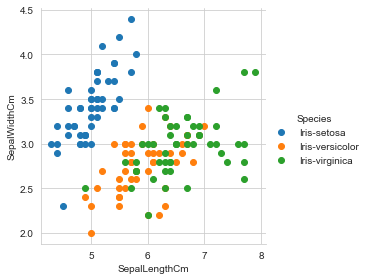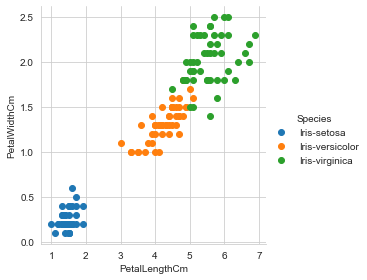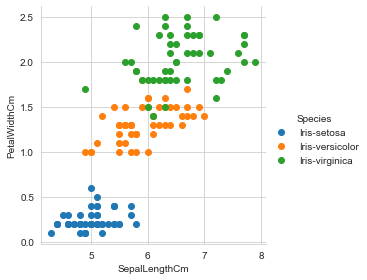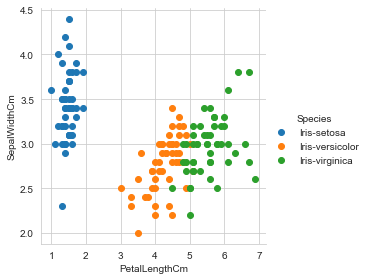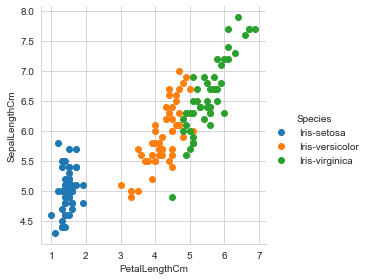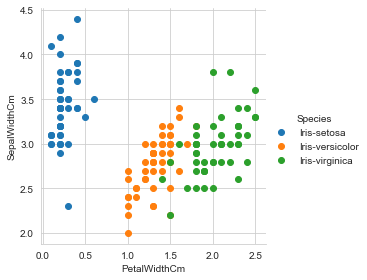## Cumulative distribution function:-

iris_setosa = iris_data.loc[iris_data["Species"] == "Iris-setosa"];

iris_virginica = iris_data.loc[iris_data["Species"] == "Iris-virginica"];

iris_versicolor = iris_data.loc[iris_data["Species"] == "Iris-versicolor"];

counts, bin_edges = np.histogram(iris_setosa['PetalLengthCm'], bins=10, density = True)

pdf = counts/(sum(counts))

print(pdf);

print(bin_edges);

cdf = np.cumsum(pdf)

plt.grid()

plt.plot(bin_edges[1:], pdf);

plt.plot(bin_edges[1:], cdf)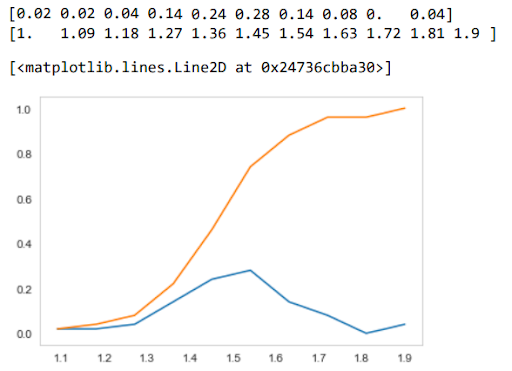## Conclusion:-

• There is a substantial correlation between petal length and width.

• Given its tiny features, we can distinguish the setosa species effortlessly.

• The Versicolor and Virginica species are frequently combined and can be difficult to distinguish. Versicolor has average characteristics while Virginica has greater.

The Iris dataset is a great application showing EDA's potential. There are more exciting applications like this one that help you understand data science in a better way. The more you practice these by yourself, the stronger your fundamentals will get.

If you have an interest in data science then enrolling in a course is the way to go. The Advanced Data Science and AI program is an amazing course for an in-depth study of data science. You will be at an advantage studying data science through experienced mentors in this program.